# 利用ggplot2画出各种漂亮图片详细教程

11173,733

## 《使用ggplot2画图》

### 1、Why use ggplot2

ggplot2是我见过最human friendly的画图软件，这得益于Leland Wilkinson在他的著作《The Grammar of Graphics》中提出了一套图形语法，把图形元素抽象成可以自由组合的成分，Hadley Wickham把这套想法在R中实现。

ggplot2基本要素

• 数据（Data）和映射（Mapping）
• 几何对象（Geometric）
• 标尺（Scale）
• 统计变换（Statistics）
• 坐标系统（Coordinante）
• 图层（Layer）
• 分面（Facet）
• 主题（Theme）

### 2、数据（Data）和映射（Mapping)

```require(ggplot2)
data(diamonds)
set.seed(42)
small <- diamonds[sample(nrow(diamonds), 1000), ]
```##       carat       cut color clarity depth table price    x    y    z
## 49345  0.71 Very Good     H     SI1  62.5    60  2096 5.68 5.75 3.57
## 50545  0.79   Premium     H     SI1  61.8    59  2275 5.97 5.91 3.67
## 15434  1.03     Ideal     F     SI1  62.4    57  6178 6.48 6.44 4.03
## 44792  0.50     Ideal     E     VS2  62.2    54  1624 5.08 5.11 3.17
## 34614  0.27     Ideal     E     VS1  61.6    56   470 4.14 4.17 2.56
## 27998  0.30   Premium     E     VS2  61.7    58   658 4.32 4.34 2.67```
`summary(small)`
```##      carat              cut      color      clarity        depth
##  Min.   :0.220   Fair     : 28   D:121   SI1    :258   Min.   :55.2
##  1st Qu.:0.400   Good     : 88   E:186   VS2    :231   1st Qu.:61.0
##  Median :0.710   Very Good:227   F:164   SI2    :175   Median :61.8
##  Mean   :0.819   Premium  :257   G:216   VS1    :141   Mean   :61.7
##  3rd Qu.:1.070   Ideal    :400   H:154   VVS2   : 91   3rd Qu.:62.5
##  Max.   :2.660                   I:106   VVS1   : 67   Max.   :72.2
##                                  J: 53   (Other): 37
##      table          price             x              y
##  Min.   :50.1   Min.   :  342   Min.   :3.85   Min.   :3.84
##  1st Qu.:56.0   1st Qu.:  990   1st Qu.:4.74   1st Qu.:4.76
##  Median :57.0   Median : 2595   Median :5.75   Median :5.78
##  Mean   :57.4   Mean   : 4111   Mean   :5.79   Mean   :5.79
##  3rd Qu.:59.0   3rd Qu.: 5495   3rd Qu.:6.60   3rd Qu.:6.61
##  Max.   :65.0   Max.   :18795   Max.   :8.83   Max.   :8.87
##
##        z
##  Min.   :2.33
##  1st Qu.:2.92
##  Median :3.55
##  Mean   :3.57
##  3rd Qu.:4.07
##  Max.   :5.58
##```

`p <- ggplot(data = small, mapping = aes(x = carat, y = price))`

`p + geom_point()`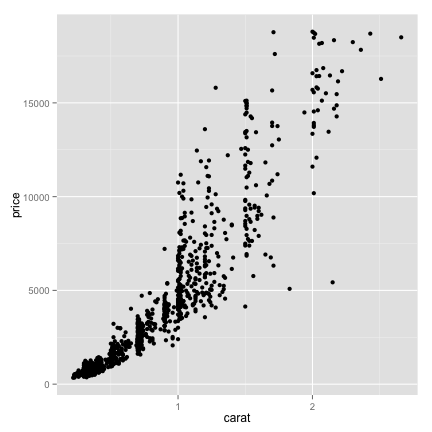```p <- ggplot(data=small, mapping=aes(x=carat, y=price, shape=cut))
p+geom_point()```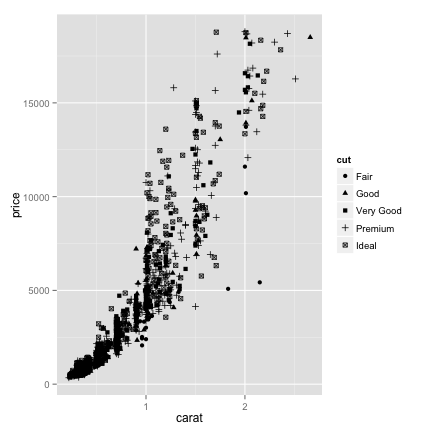```p <- ggplot(data=small, mapping=aes(x=carat, y=price, shape=cut, colour=color))
p+geom_point()```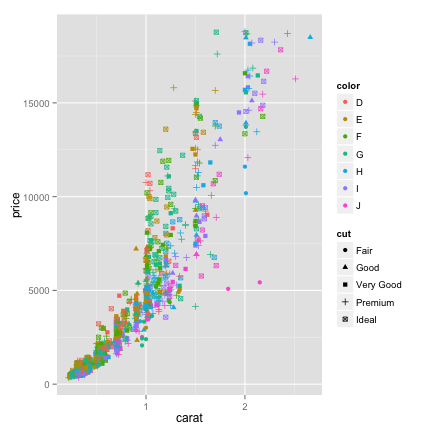### 3、几何对象（Geometric）

geom_point()完成的就是几何对象的映射，ggplot2提供了各种几何对象映射，如geom_histogram用于直方图，geom_bar用于画柱状图，geom_boxplot用于画箱式图等等。

```p <- ggplot(small)
p+geom_point(aes(x=carat, y=price, shape=cut, colour=color))```

ggplot2支持图层，我通常把不同的图层中共用的映射提供给ggplot函数，而某一几何对象才需要的映射参数提供给geom_xxx函数。

#### 直方图

`ggplot(small)+geom_histogram(aes(x=price))`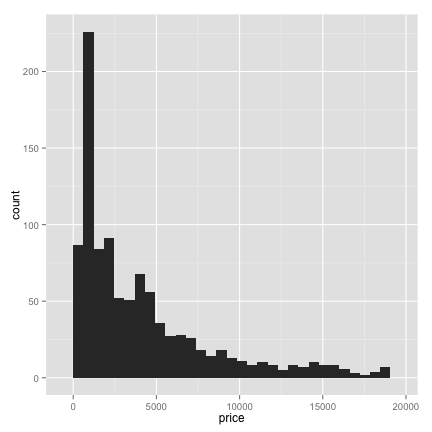`ggplot(small)+geom_histogram(aes(x=price, fill=cut))`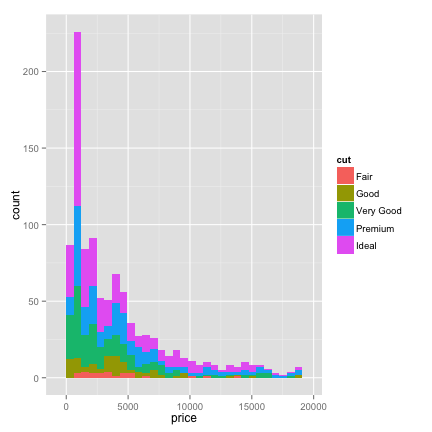`ggplot(small)+geom_histogram(aes(x=price, fill=cut), position="dodge")`

`ggplot(small)+geom_histogram(aes(x=price, fill=cut), position="fill")`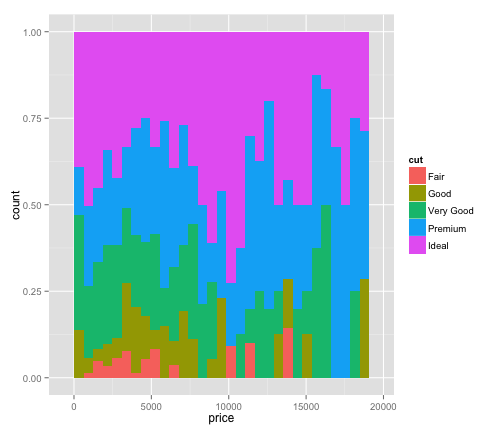#### 柱状图

`ggplot(small)+geom_bar(aes(x=clarity))`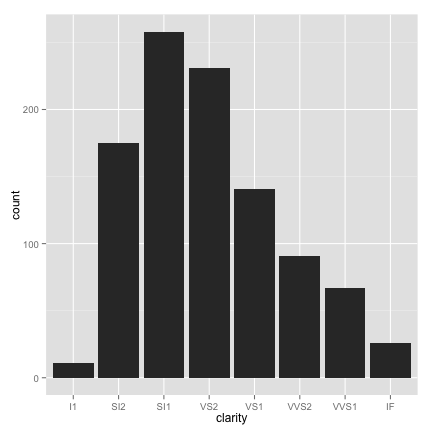`ggplot()+geom_bar(aes(x=c(LETTERS[1:3]),y=1:3), stat="identity")`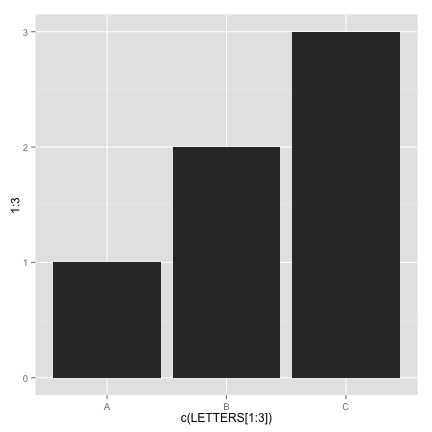#### 密度函数图

`ggplot(small)+geom_density(aes(x=price, colour=cut))`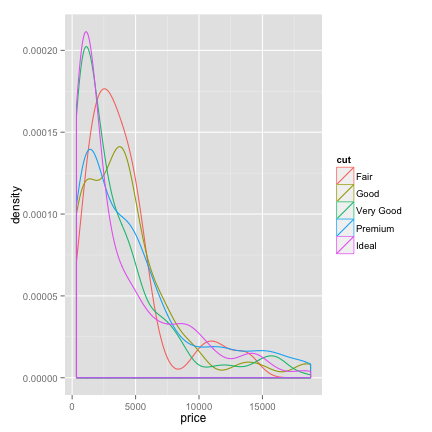`ggplot(small)+geom_density(aes(x=price,fill=clarity))`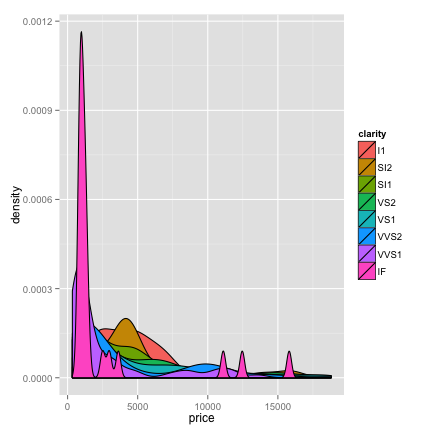colour参数指定的是曲线的颜色，而fill是往曲线下面填充颜色。

`ggplot(small)+geom_boxplot(aes(x=cut, y=price,fill=color))`

geom_boxplot将数据映射到箱式图上，上面的代码，我们应该很熟悉了，按切工(cut)分类，对价格(price)变量画箱式图，再分开按照color变量填充颜色。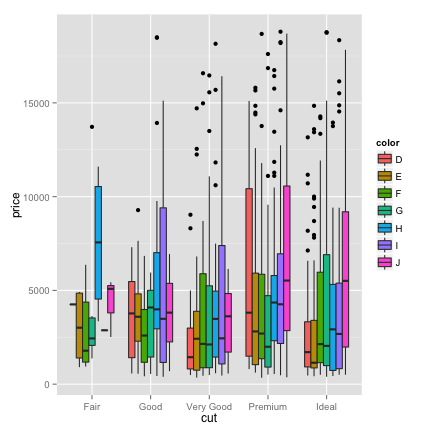ggplot2提供了很多的geom_xxx函数，可以满足我们对各种图形绘制的需求。

```geom_abline 	geom_area
geom_bar 		geom_bin2d
geom_blank 		geom_boxplot
geom_contour 	geom_crossbar
geom_density 	geom_density2d
geom_dotplot 	geom_errorbar
geom_errorbarh 	geom_freqpoly
geom_hex 		geom_histogram
geom_hline 		geom_jitter
geom_line 		geom_linerange
geom_map 		geom_path
geom_point 		geom_pointrange
geom_polygon 	geom_quantile
geom_raster 	geom_rect
geom_ribbon 	geom_rug
geom_segment 	geom_smooth
geom_step 		geom_text
geom_tile 		geom_violin
geom_vline```

### 4、标尺（Scale）

`ggplot(small)+geom_point(aes(x=carat, y=price, shape=cut, colour=color))+scale_y_log10()+scale_colour_manual(values=rainbow(7))`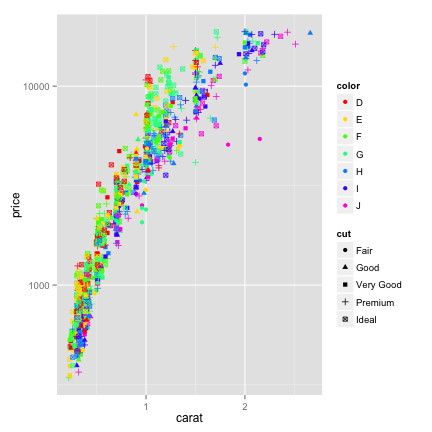### 5、统计变换（Statistics）

`ggplot(small, aes(x=carat, y=price))+geom_point()+scale_y_log10()+stat_smooth()`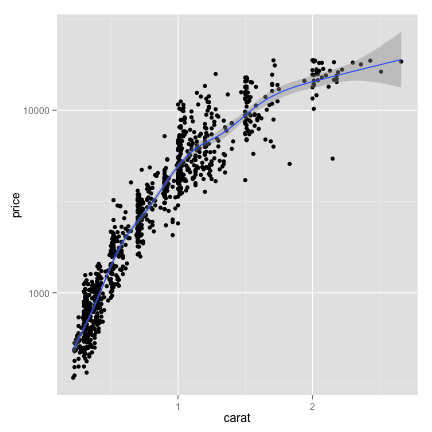ggplot2提供了多种统计变换方式：

```stat_abline       stat_contour      stat_identity     stat_summary
stat_bin          stat_density      stat_qq           stat_summary2d
stat_bin2d        stat_density2d    stat_quantile     stat_summary_hex
stat_bindot       stat_ecdf         stat_smooth       stat_unique
stat_binhex       stat_function     stat_spoke        stat_vline
stat_boxplot      stat_hline        stat_sum          stat_ydensity```

### 6、坐标系统（Coordinante）

`ggplot(small)+geom_bar(aes(x=cut, fill=cut))+coord_flip()`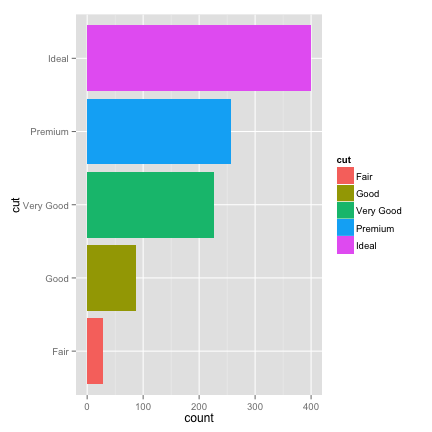`ggplot(small)+geom_bar(aes(x=factor(1), fill=cut))+coord_polar(theta="y")`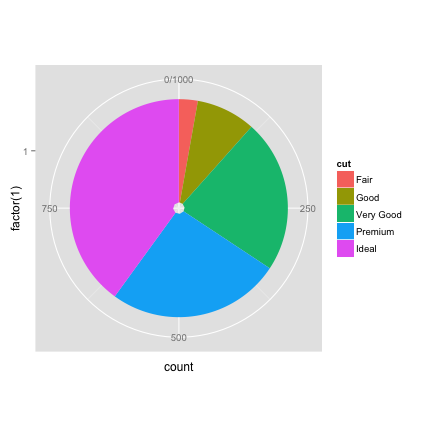`ggplot(small)+geom_bar(aes(x=factor(1), fill=cut))+coord_polar()`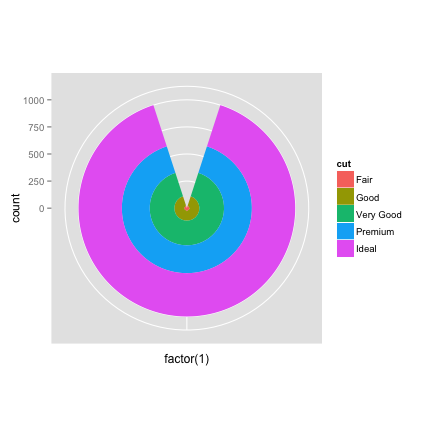`ggplot(small)+geom_bar(aes(x=clarity, fill=cut))+coord_polar()`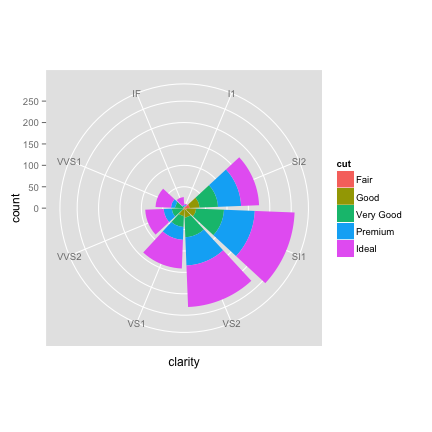### 7、图层（Layer）

photoshop流行的原因在于PS 3.0时引入图层的概念，ggplot的牛B之处在于使用+号来叠加图层，这堪称是泛型编程的典范。

```require(ggplot2)
f1data.frame(x=x,y=y)
d -3*sqrt(33)/7,]
return(d)
}

x1data.frame(x2=x2, y2=y2)
p2data.frame(x3=x3, y3=y3)
p3data.frame(x4=x4,y4=y4)
p4data.frame(x5=x5,y5=y5)
p5data.frame(x6=x6,y6=y6)
p6```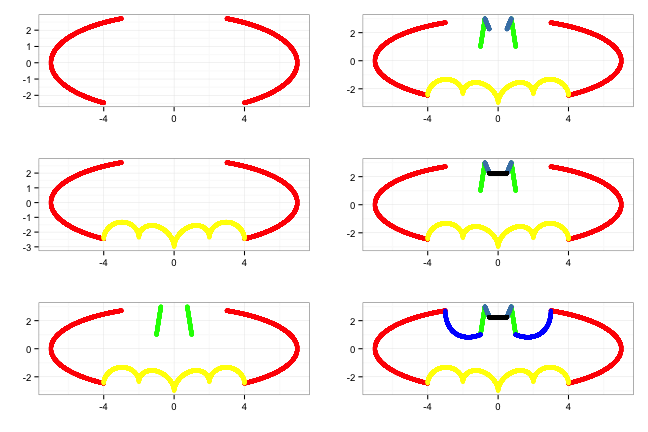```Normaldata.frame(V=c("Normal", "Cancer"), mean=m, sd=s)
d\$V```

### 8、分面（Facet）

`ggplot(small, aes(x=carat, y=price))+geom_point(aes(colour=cut))+scale_y_log10() +facet_wrap(~cut)+stat_smooth()`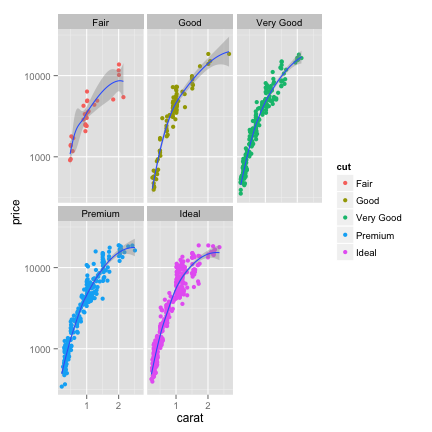### 9、主题（Theme）

`p`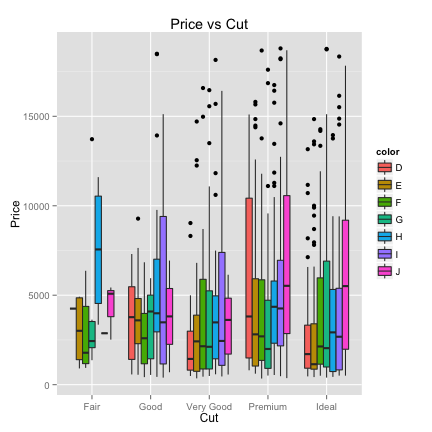ggplot2提供一些已经写好的主题，比如theme_grey()为默认主题，我经常用的theme_bw()为白色背景的主题，还有theme_classic()主题，和R的基础画图函数较像。

```theme_economist theme_economist_white
theme_wsj 	 	theme_excel
theme_few 	 	theme_foundation
theme_igray 	theme_solarized
theme_stata 	theme_tufte```
```require(ggthemes)
p + theme_wsj()```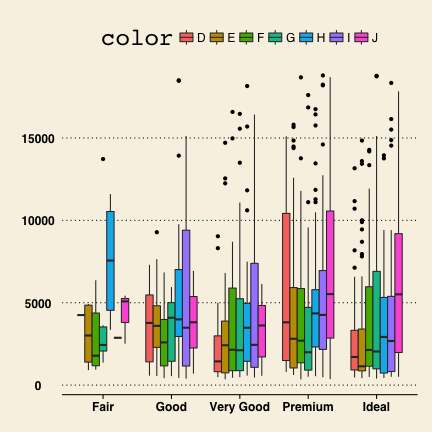```fdata.frame(x=x,y=y)

p```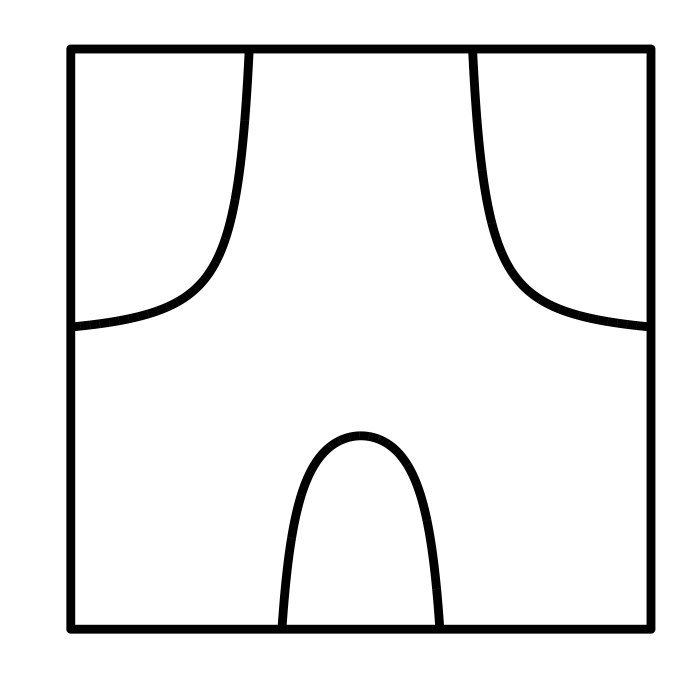### 10、二维密度图

`ggplot(diamonds, aes(carat, price))+ stat_density2d(aes(fill = ..level..), geom="polygon")+ scale_fill_continuous(high='darkred',low='darkgreen')`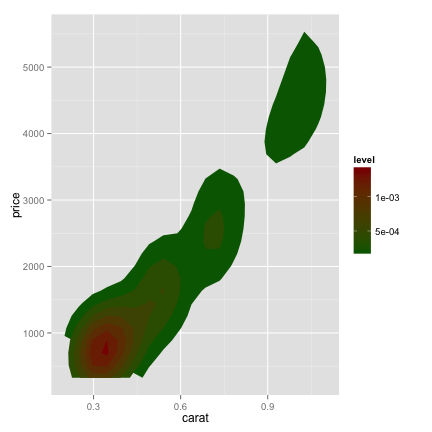### 11、ggplot2实战

```fdata.frame(x=c(x1,x2,x3), y=rep(y,3), type=rep(LETTERS[1:3], each=length(y)))
p```

```theta data.frame(x=radius*sin(theta), y=radius*cos(theta))
ggplot(dd, aes(x, y))+geom_path()+theme_null()+xlab("")+ylab("")```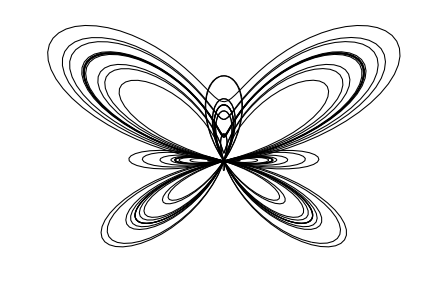• 本文由 发表
• 转载请务必保留本文链接：https://www.plob.org/article/7264.html

• ygc 1

专业转载员还要改我的标题！！！

• ybzhao

@ ygc Guangchuang兄请见谅，原标题已经在文章中注明。您这篇文章实在是写的太好了，我看完之后觉得朴实的标题无法体现丰富的内容，所以plob转载的时候换了个标题。另一方面是让这篇文章与plob上其他短篇的ggplot2文章区分，从而更多plob网友注意到这篇文章，并从中获益。

plob的定位就是收集和整理生物信息学相关各种资源，创建生物信息资源文库，尤其是technique方面的。网站资源收集自各个网友的博客，同时有部分内容是包括我在内的plob网友原创并第一时间发布在plob上。

plob的发展还需要像您这样的高手的指点和支持啊。

• ygc 1

这个网站值得支持，是中文生信的知识库啊 ：）

• zhhu 0

@ ygc 老师：有个问题请教一下，您的博文说到,箱式图
数据量比较大的时候，用直方图和密度函数图是表示数据分布的好方法，而在数据量较少的时候，比如很多的生物实验，很多时候大家都是使用柱状图+errorbar的形式来表示，不过这种方法的信息量非常低，被Nature Methods吐槽，这种情况推荐使用boxplot。我想问一下，boxplot对数据有什么要求？

• ygc 0

@ zhhu 要问问题，请稳步到原博文。

• snailQH 1

写的很好！赞一个！

• meilirenshengzcs 0

很精彩，我想知道在哪里可以下栽ggplot 的安装包？

• zhangmeng 0

require(ggplot2)
values=c(888672, 130436, 243200, 3680578, 263503,162848,61548,123663,14241)
labels=c(“A”,”C”,”B”,”D”,”E”,”F”,”G”,”H”,”I”)
colours=c(“#8dd3c7”, “#ffffb3”, “#bebada”, “#80b1d3”, “#fb8072”, “#fdb462”, “#b3de69”, “#d9d9d9”, “#fccde5”)
percent_str <- paste(round(values/sum(values) * 100,1), "%", sep="")
values <- data.frame(Percent = round(values/sum(values) * 100,1), Type = labels,percent=percent_str )
pie <- ggplot(values, aes(x = "" ,y = percent, fill = Type)) + geom_bar(width = 3)
pie = pie + coord_polar("y")
pie = pie + xlab('') + ylab('') + labs(fill="Types")
#pie + scale_fill_manual(values = colours)
pie + scale_fill_manual(values = colours,labels = labels)
pie <- ggplot(values, aes(x = "x_list" ,y = Percent, fill = Type)) + geom_bar(width = 3)

一直报错提示：Error: stat_count() must not be used with a y aesthetic.
所以想问问老师，是什么地方的出错？
非常感谢！

• zhuwen96485 1

真心觉得不错，感谢分享

• Olivia 0

非常好,值得学习！强烈推荐